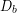# 12.2: Unit 4 Lab- Hydrostatic Weighing

$$\newcommand{\vecs}{\overset { \rightharpoonup} {\mathbf{#1}} }$$ $$\newcommand{\vecd}{\overset{-\!-\!\rightharpoonup}{\vphantom{a}\smash {#1}}}$$$$\newcommand{\id}{\mathrm{id}}$$ $$\newcommand{\Span}{\mathrm{span}}$$ $$\newcommand{\kernel}{\mathrm{null}\,}$$ $$\newcommand{\range}{\mathrm{range}\,}$$ $$\newcommand{\RealPart}{\mathrm{Re}}$$ $$\newcommand{\ImaginaryPart}{\mathrm{Im}}$$ $$\newcommand{\Argument}{\mathrm{Arg}}$$ $$\newcommand{\norm}{\| #1 \|}$$ $$\newcommand{\inner}{\langle #1, #2 \rangle}$$ $$\newcommand{\Span}{\mathrm{span}}$$ $$\newcommand{\id}{\mathrm{id}}$$ $$\newcommand{\Span}{\mathrm{span}}$$ $$\newcommand{\kernel}{\mathrm{null}\,}$$ $$\newcommand{\range}{\mathrm{range}\,}$$ $$\newcommand{\RealPart}{\mathrm{Re}}$$ $$\newcommand{\ImaginaryPart}{\mathrm{Im}}$$ $$\newcommand{\Argument}{\mathrm{Arg}}$$ $$\newcommand{\norm}{\| #1 \|}$$ $$\newcommand{\inner}{\langle #1, #2 \rangle}$$ $$\newcommand{\Span}{\mathrm{span}}$$$$\newcommand{\AA}{\unicode[.8,0]{x212B}}$$

## Hydrostatic Weighing

### Materials:

• graduated cylinder (required for displacement method, but not required for hydrostatic weighing method)
• plastic bin to contain spills
• object to submerge
• calculator
• scale with at least 0.1 gram precision
• for distance learners, access to online forums, videos, and help features for the spreadsheet software will likely be necessary
• force sensor + computer with control and analysis software OR spring scale with 0.1 gram precision (required for hydrostsatic weighing method, but not for required for displacement method)

### Objective

The objective of our lab is to determine the body density of an object in the same way we would for a person. We will determine body density in two ways, one being the displacement method and the other being hydrostatic weighing.

You might want to watch this video to refresh your memory regarding the general process for hydrostatic weighing:A YouTube element has been excluded from this version of the text. You can view it online here: https://openoregon.pressbooks.pub/bodyphysics/?p=1370

### General Methods

The video above does not explain how to actually calculate the body fat percentage (BF%) from the hydrostatic weighing data. The BF% calculation is done using rather complex formulas that require require body density,, as an input:Formulas for calculating body fat percentage from body density and other body measurements. Image Credit: MattVerlinich via InstructablesFigure: For fun we will use the body density value we find as an input to calculate the “body fat percentage” of our object, but of course this number will not be meaningful because the final BF% formula we are using is an empirical model based only on data from people.

### Hydrostatic Weighing Method (require force sensor or spring scale)

Measure the body weight of your object in air and record here:___________

If your measurement device provided you with mass instead of weight, then calculate the object weight. You may need to look up the equation relating mass and weight of an object near the surface of the Earth. Show your work.

Submerge the object and measure the under water weight, also known as the apparent weight. Record here:___________

Again, if your measurement device provided you with mass instead of weight, then calculate the object weight. Show your work.

Based on your measured weight and apparent weight, what must be the size of the buoyant force?

Use Archimedes’ Principle to determine the weight of the water displaced by the object. Explain your reasoning.

Calculate the mass of the water displaced. You may need to look up the equation relating mass and weight of an object near the surface of the Earth. Show your work.

Look up the density of water and record here:________

Now use the definition of density to find the volume of the displaced water. Show your work.

How does the volume of displaced water compare to the volume of the object? Explain your reasoning (remember, the object was completely submerged).

Now you know the body volume of the object, so if you know its body mass then you can calculate its body density. If you already have body mass from an earlier measurement calculate the body density. If you only know body weight from an earlier measurement then calculate the body mass from body weight, then calculate body density. Show all work.

Does the formula result agree with your previous result?

### Displacement Method (requires graduated cylinder)

Measure the body mass of your object and record here:__________

If your measurement device only provided you with body weight, then calculate the body mass. You may need to look up the equation relating mass and weight of an object near the surface of the Earth. Show your work.

Submerge your object in the graduated cylinder and measure the change in volume. This is the volume of water displaced by the object.

Record displaced volume here:__________

How does the volume of displaced water compare to the volume of the object? Explain your reasoning (remember, the object was completely submerged).